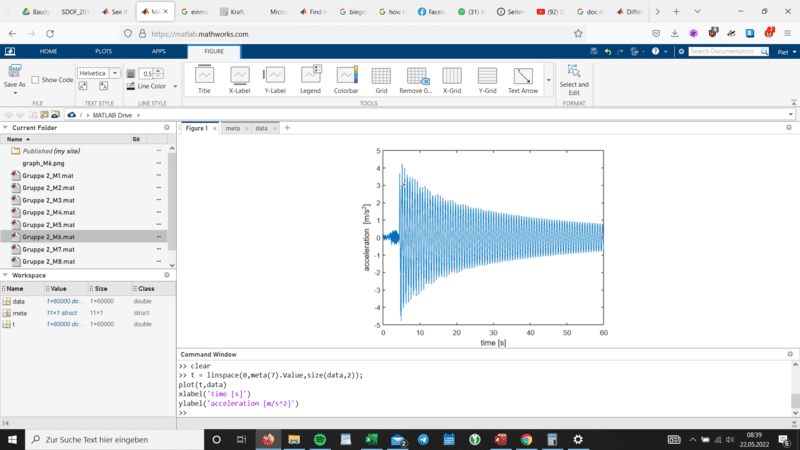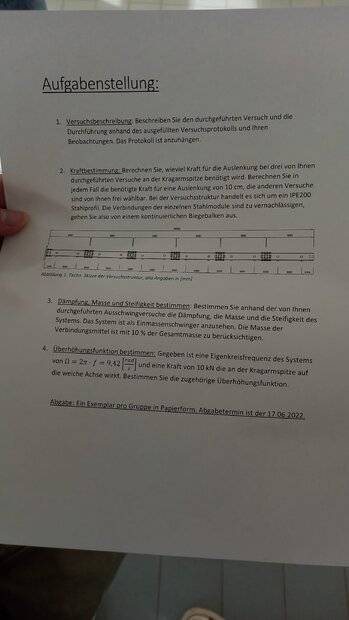# Need help in solving SDOF cantilever

• Engineering
pjotrjanusz
Homework Statement:
Calculate force, mass, damping and stiffness of horicontally oscillated IPE beam, completely fixed on the one end and free on the other
Length: 5990mm ;Deflection: 1cm, 3cm, 5cm, 7cm
Relevant Equations:
I am given data of our project that includes the measurement of the acceleration of the beam after being deflected as named above each and left to swing for 1 minute.
1.) I'd calculate the force needed to deflect the beam by using the method of virtual work for t=0 which is u[L] = FL^3/3EI. From there on I am completely clueless.
2.) Task is to calculate damping, mass and stiffness of the SDOF by using the data sheets which is basicly the u''(t) function of each experiment. I thought the mass of the SDOF can be generalised by putting the mass of the IPE beam (V*p) concentrated in a singular dot which represents the SDOF. Am I wrong by assuming this?
3.) Bending-stiffness in statics is always a combination of young's modulus and moment of inertia, but how do I find out the stiffness the beam has when experiencing dynamic stress? I don't know how to calculate the stiffness.
4.) I'd calculate the damping of the system by solving the differential equation of motion. But how am I supposed to get the inputs for that equations?
-> Basically I am confused because we are only given the acceleration graphs of the tip of that beam after being deflected by us. I just need a good tip on how to start. Do I have to select some critical coordinates in those graphs and use them as input for the ODE to or three times as damping and stiffness is going to be constant anyways? each of the 8 graphs contain more than 60k coordinates... You can cleary see how lost I am so I'd be so thankfull for any help or tips!Last edited:

Mentor
Have you learned that continuous beams have an infinite number of natural frequencies? And that a SDOF model applies to only one of those natural frequencies, normally the lowest frequency? The confusion seems to be that a SDOF model has three parameters, and you have 60,000 data points. These type of problems are solved by curve fitting.

From there on I am completely clueless.
Well, here are some clues:
1) The data plot shows the natural frequency, and the damping rate.
2) Natural frequency of a SDOF system is the ratio of stiffness to mass.
3) You can measure the stiffness directly by pulling on the beam with a known force and measuring the deflection with a ruler.
4) That allows you to calculate the mass of a SDOF with the same natural frequency of the beam. That mass will not be equal to the mass of the beam. Do you know why?
5) Now that you have stiffness and mass, find the damping that matches the rate of damping in the data. Plot the response of the resulting equation on the same axes as your experimental data. They should match.

•berkeman